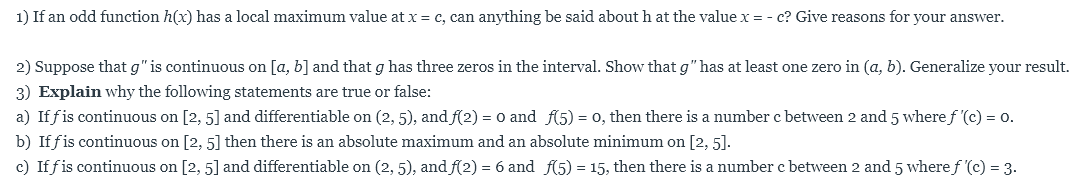# 1) If an odd function h(x) has a local maximum value at x c, can anything be said about h at the value x = - c? Give reasons for your answer.2) Suppose that g" is continuous on [a, b] and that g has three zeros in the interval. Show that g" has at least one zero in (a, b). Generalize your result.3) Explain why the following statements are true or false:a) If fis continuous on [2, 5] and differentiable on (2, 5), and f(2)o, then there is a number c between 2 and 5 where f '(c)o and f5)o.=b) Iffis continuous on [2, 5] then there is an absolute maximum and an absolute minimum on [2, 5].c) Iffis continuous on [2, 5] and differentiable on (2, 5), andf(2) = 6 and f(5) = 15, then there is a number c between 2 and 5 wheref '(c) = 3.

Question
80 viewshelp_outlineImage Transcriptionclose1) If an odd function h(x) has a local maximum value at x c, can anything be said about h at the value x = - c? Give reasons for your answer. 2) Suppose that g" is continuous on [a, b] and that g has three zeros in the interval. Show that g" has at least one zero in (a, b). Generalize your result. 3) Explain why the following statements are true or false: a) If fis continuous on [2, 5] and differentiable on (2, 5), and f(2) o, then there is a number c between 2 and 5 where f '(c) o and f5) o. = b) Iffis continuous on [2, 5] then there is an absolute maximum and an absolute minimum on [2, 5]. c) Iffis continuous on [2, 5] and differentiable on (2, 5), andf(2) = 6 and f(5) = 15, then there is a number c between 2 and 5 wheref '(c) = 3. fullscreen
check_circle

Step 1

We’ll answer the first question since the exact one wasn’t specified. Please submit a new questio...

### Want to see the full answer?

See Solution

#### Want to see this answer and more?

Solutions are written by subject experts who are available 24/7. Questions are typically answered within 1 hour.*

See Solution
*Response times may vary by subject and question.
Tagged in

### Calculus